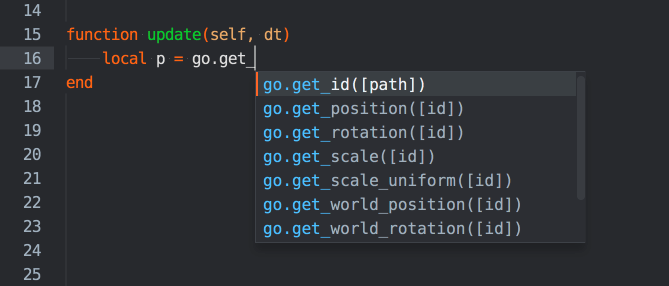# 脚本

## 脚本类型

Defold 里有三种脚本, 每种脚本对应各自的 Defold 函数库.

Logic scripts

GUI scripts

Render scripts

## 脚本运行, 回调和 self

Defold 把 Lua 脚本作为引擎生命周期的一部分来执行并且向脚本暴露了一些生命周期函数. 当你把脚本组件附加到游戏对象上时这个脚本就变成了游戏对象及其组件的生命周期的一部分. 脚本加载后先进行上下文评估, 然后引擎开始执行以下函数同时传递一个当前组件实例的引用作参数. 这个参数就是 `self` , 可以用来保存组件实例上的各种状态.

`self` 是一个 userdata 对象, 可以用作 Lua 表但是不能使用 `pairs()` 或者 `ipairs()`迭代, 而且也不能使用 `pprint()` 输出其内容.

`init(self)`

``````function init(self)
-- 这些变量会在组件生命周期中一直存在
self.my_var = "something"
self.age = 0
end
``````
`final(self)`

``````function final(self)
if self.my_var == "something" then
-- 做一些清理工作
end
end
``````
`update(self, dt)`

``````function update(self, dt)
self.age = self.age + dt -- 把每帧时差加到 age 上
end
``````
`fixed_update(self, dt)`

``````function fixed_update(self, dt)
msg.post("#co", "apply_force", {force = vmath.vector3(1, 0, 0), position = go.get_world_position()})
end
``````
`on_message(self, message_id, message, sender)`

``````  function on_message(self, message_id, message, sender)
if message_id == hash("increase_score") then
self.total_score = self.total_score + message.score
end
end
``````
`on_input(self, action_id, action)`

``````  function on_input(self, action_id, action)
if action_id == hash("touch") and action.pressed then
print("Touch", action.x, action.y)
end
end
``````
`on_reload(self)`

``````function on_reload(self)
print(self.age) -- 输出对象的 age
end
``````

## 链式逻辑

`update()` 函数中可以实现复杂的行为定义比如每帧运行的状态机—有时这是不错的用法. 但是每帧调用一个 `update()` 一点点计时有点浪费. 可以的话最好自己实现一个不依靠update 链式逻辑. 它不是被动等待时间累计而是主动设置触发时间目标. 此外, 链式逻辑的设计往往需要尽量简洁稳定易于实现.

``````function init(self)
-- 计时器.
self.counter = 0
-- 状态.
self.state = "first"
end

function update(self, dt)
self.counter = self.counter + dt
if self.counter >= 2.0 and self.state == "first" then
-- 2 秒后发送消息
msg.post("some_object", "some_message")
self.state = "waiting"
end
if self.counter >= 5.0 and self.state == "second" then
-- 状态改变后 5 秒再发送一个消息
msg.post("another_object", "another_message")
--再次改变状态以避免再次进入这里的代码.
self.state = nil
end
end

function on_message(self, message_id, message, sender)
if message_id == hash("response") then
-- 第一个消息收到, 改变状态
self.state = "second"
-- 清空计时器
self.counter = 0
end
end
``````

``````local function send_first()
msg.post("some_object", "some_message")
end

function init(self)
-- 等 2 秒后调用 send_first()
timer.delay(2, false, send_first)
end

local function send_second()
msg.post("another_object", "another_message")
end

function on_message(self, message_id, message, sender)
if message_id == hash("response") then
-- 等 5 秒后调用 send_second()
timer.delay(5, false, send_second)
end
end
``````

## 编辑器支持

Defold 编辑器支持 Lua 脚本编辑, 还提供语法高亮和自动补全功能. 要让 Defold 补全函数名, 按 Ctrl+Space 会弹出相关函名数列表.Did you spot an error or do you have a suggestion? Please let us know on GitHub!